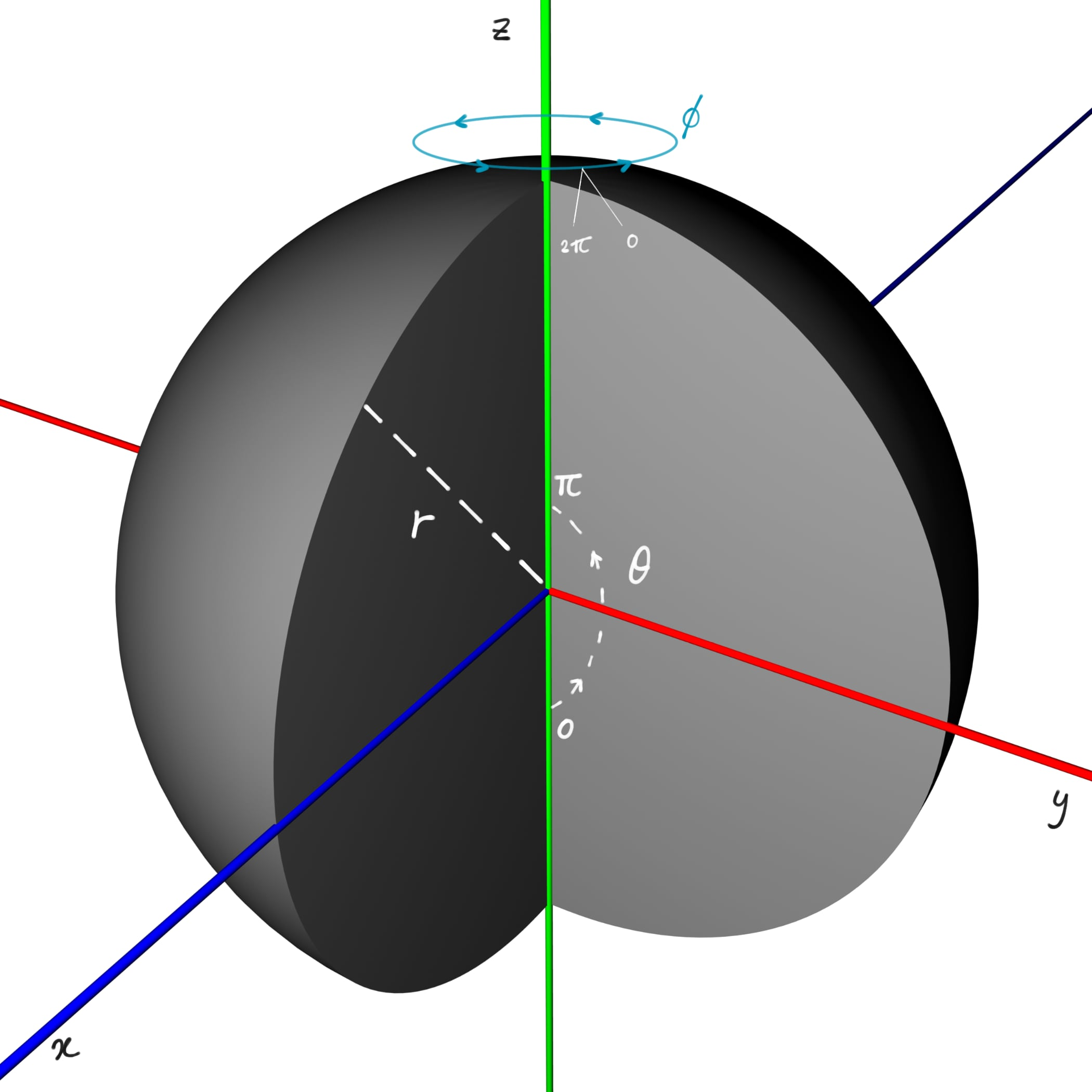# Deriving the volume of the inside of a sphere using spherical coordinates

Even though the well-known Archimedes has derived the formula for the inside of a sphere long before we were born, its derivation obtained through the use of spherical coordinates and a volume integral is not often seen in undergraduate textbooks.

In this post, we will derive the following formula for the volume of a ball:

\begin{equation}
V = \frac{4}{3}\pi r^3,
\end{equation}

where $r$ is the radius.

Note the use of the word ball as opposed to sphere; the latter denotes the infinitely thin shell, or, surface, of a perfectly round geometrical object in three-dimensional space. A surface has no volume, hence, we prefer to refer to it as a ball.

This could be seen as a second-year university-level post.

### Spherical coordinates

The volume of a cuboid $\delta V$ with length $a$, width $b$, height $c$ is given by $\delta V = a \times b \times c$.

In Figure 1, you see a sketch of a volume element of a ball. Although its edges are curved, to calculate its volume, here too, we can use

\begin{equation}
\delta V \approx a \times b \times c,
\end{equation}

even though it is only an approximation.

To use spherical coordinates, we can define $a$, $b$, and $c$ as follows:
\begin{align}
a &= PQ\delta\phi = r\sin\theta \, \delta\phi, \\
b &= r\delta\theta, \\
c &= \delta r.
\end{align}

So, equation (2) becomes

\begin{align}
\delta V &\approx r\sin\theta \, \delta\phi \times r\delta\theta \times \delta r, \nonumber \\
&\approx r^2\sin\theta \, \delta\phi \, \delta\theta \, \delta r.
\end{align}

### Volume integral

Note that the relation becomes more precise when $\delta\phi$, $\delta\theta$, and $\delta r$ tend to zero. So, we can now write the volume integral for our ball $B$ as follows:

\begin{equation*}
V_B = \int_B dV_B = \int_\phi \int_\theta \int_r r^2\sin\theta \, dr \, d\theta \, d\phi.
\end{equation*}Figure 2: To integrate over the infinite number of points (inside and on the surface) of a ball, one angle varies from $0$ to $2\pi$, which is $\phi$, in this case. Angle $\theta$ only needs to vary half of that as a ball is rotationally symmetric. Of course, bound by radius $r$.

To set the upper and lower bounds for our integrals, we note that a ball has rotational symmetry about the $z$-axis (besides infinitely many others through the centre too). We will exploit this. We refer to Figure 2.

Firstly, to integrate over infinitely many points between $0$ and $r$, the lower bound is $0$ and the upper bound is $r$:

\begin{equation*} V_B = \int_B dV_B = \int_\phi \int_\theta \int_{r=0}^r r^2\sin\theta \, dr \, d\theta \, d\phi.
\end{equation*}

Secondly, to integrate over infinitely many points in the plane of angle $\theta$, we only need to regard the angles between $0$ and $\pi$,

\begin{equation*}
V_B = \int_B dV_B = \int_\phi \int_{\theta=0}^{\theta=\pi} \int_{r=0}^r r^2\sin\theta \, dr \, d\theta \, d\phi,
\end{equation*}

as we will proceed to, thirdly, rotate this plane, as it were, about the $z$-axis to integrate over infinitely many planes about said axis, which complete the shape of our ball. Hence, $\phi$ varies between $0$ and $2\pi$.

And so, we calculate

\begin{align}
V_B = \int_B dV_B &= \int_{\phi=0}^{\phi=2\pi} \int_{\theta=0}^{\theta=\pi} \int_{r=0}^r r^2\sin\theta \, dr \, d\theta \, d\phi, \\
&= \int_{\phi=0}^{\phi=2\pi} \int_{\theta=0}^{\theta=\pi} \left(\frac{1}{3} r^3\sin\theta \Big|_0^r\right) d\theta \, d\phi, \nonumber \\
&= \frac{1}{3} \int_{\phi=0}^{\phi=2\pi} \int_{\theta=0}^{\theta=\pi} r^3\sin\theta \, d\theta \, d\phi, \nonumber \\
&= -\frac{1}{3} \int_{\phi=0}^{\phi=2\pi} \left( r^3\cos\theta \Big|_0^{\pi} \right) \, d\phi, \nonumber \\
&= \frac{2}{3} \int_{\phi=0}^{\phi=2\pi} r^3 \, d\phi, \nonumber \\
&= \frac{2}{3} \left( \phi r^3 \Big|_0^{2\pi} \right), \nonumber \\
&= \frac{4}{3}\pi r^3,
\end{align}

which is the desired result equal to equation (1).